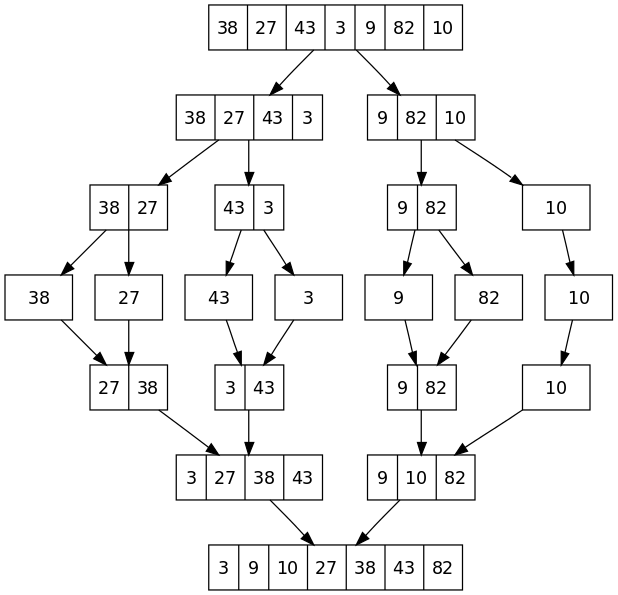# Implementation of Merge Sort with C# and analysis of running time on best, worst and average case

 Category: Data Structure And Algorithms Tags:Merge Sort Code Files

## Introduction

Merge Sort is one of the efficient sorting algorithm sorts n elements of an array in n log n running time. Merge sort likely Insertion Sort can be explained by card game.

Let's suppose we have 2 piles of cards faced up on table, both are sorted and smallest on top. Now we have to combine them to make a single pile which should be card faces down and sorted. Here what we can do is we can remove one card from both of piles and place them in new output pile comparing smaller one should be placed first and if any one input pile is finished first, we just have to place other input pile on output pile.

The merge sort works on divide and conquer rule where first it breaks problem in small chunks then sort them and then merge them back.Figure Reference: Wikipedia

## Implementation

To implement merge sort we will use C#, but at this level programming language doesn't matter, you can use c, c++, java etc

```public class MergeSort{    int[] A; // Array has to be sorted    public MergeSort(int[] array) //constructor    {        A = array;    }    /// <summary>    /// Sorts in Increasing order    /// </summary>    /// <returns>Sorted Array</returns>    public int[] Sort()    {        Devide(0, (A.Length - 1));        return A;    }    /// <summary>    /// Devides the array    /// </summary>    /// <param name="p">Start Index</param>    /// <param name="r">Last Index</param>    public void Devide(int p, int r)    {        if (p < r)        {            int q = (p + r) / 2; //middle is q            Devide(p, q); //devides untill left with chunk of 2 elements            Devide(q + 1, r);            Merge(p, q, r);        }    }    /// <summary>    /// Merges the sorted arrays into one    /// </summary>    /// <param name="p">Start Index</param>    /// <param name="q">Middle Index</param>    /// <param name="r">Last Index</param>    public void Merge(int p, int q, int r)    {        int n1 = q - p + 1; //required length for left temp array        int n2 = r - q;     //required length for right temp array        int[] tempLeft = new int[n1];        int[] tempRight = new int[n2];        int i = 0, j = 0;        for (i = 0; i < n1; i++)   //insering data in left array            tempLeft[i] = A[p + i];        for (j = 0; j < n2; j++)   //inserting data in right array            tempRight[j] = A[q + 1 + j];        i = 0;        j = 0;        int k;        for (k = p; i < n1 && j < n2; k++)        {            if (tempLeft[i] <= tempRight[j]) //*sorting & merging process            {                A[k] = tempLeft[i];                i++;            }            else            {                A[k] = tempRight[j];                j++;            }        }        while (i < n1) //dumping if temp left is still having data        {            A[k] = tempLeft[i];            i++;            k++;        }        while (j < n2) //dumping if temp right is still having data        {            A[k] = tempRight[j];            j++;            k++;        }    }}
```

In above code we are having an array of n elements and we are calling Divide Method to divide the array in sub-array (0 To middle) and ((middle+1) To last) and calling this function recursively until we break it in sub array of 2 max elements.

The Method Merge to sort and merge the sub-array, to achieve this we created two temporary arrays and sorted it in by comparing their elements one by one using for-loop. We run the loop until one of them is empty. If any one array is left with elements we will simply put it in resultant array.Image Reference: Wikipedia

Let's run above code:

`MergeSort mergeSort = new MergeSort(new int[] { 2, 1, 7, 4, 9, 3, 6 });var sortedArr = mergeSort.Sort();foreach (int ele in sortedArr)    Console.WriteLine(ele);Console.ReadLine();`

Output:

1

2

3

4

6

7

9

## Analysis

Let's suppose we have an array containing n elements and algorithm divides it in two problems of n/2 then again n/2 will be divided in two n/4 and so on. Let we divide the array of "n" into "a" sub problems and size of each is "n/b". So it takes T(n/b) to solve one and aT(n/b) to solve all the problems. If we take D(n) time to divide and M(n) time to merge it back then total running time will be:

T(n) = aT(n/b) + D(n) + M(n)

But dividing is just calculation of middle element that is O(1) linear time so we neglect the D(n) and if array is having even numbers each divide gives exactly n/2 elements and sorting the two n/2 elements will take time about 2T(n/2) so we can write:

T(n) = 2T(n/2) + M(n)

now writing M(n) = C n

T(n) = 2 T(n/2) + C n                ...Eq(1)

So   T(n/2) = 2 T(n/4) + C n/2           ...Eq(2)

substituting value of T(n/2) from Eq(2) to Eq(1)

T(n) = 2[2 T(n/4) + C n/2] + C n

or      T(n) = 4 T(n/4) + 2 C n

Same:

or      T(n) = 8 T(n/8) + 3 C n

or      T(n) = 2k T(n/2k) + k C n

**But for some value of k where 2k = n (or k = log2n)

so      T(n) = 2k T(1) + log(n) C n

or       T(n) = n + n log(n) C

or        T(n) = O(n log n) or better to say Θ(n log n) because lower bound is also same

So for best, worst and average case the complexity will be n log n.

 Like0 People
Last modified on 29 October 2018Nikhil Joshi Ceo & Founder at Dotnetlovers Atricles: 132 Questions: 9 Given Best Solutions: 9 *

## Comments:

No Comments Yet

You are not loggedin, please login or signup to add comments:

## Existing User

Login via:## New Userx